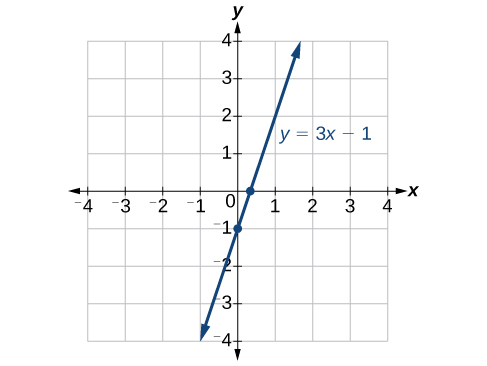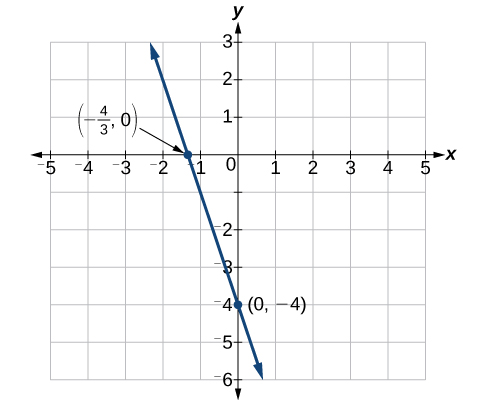## Finding x-intercepts and y-intercepts

The intercepts of a graph are points at which the graph crosses the axes. The x-intercept is the point at which the graph crosses the x-axis. At this point, the y-coordinate is zero. The y-intercept is the point at which the graph crosses the y-axis. At this point, the x-coordinate is zero.

To determine the x-intercept, we set y equal to zero and solve for x. Similarly, to determine the y-intercept, we set x equal to zero and solve for y. For example, lets find the intercepts of the equation $y=3x - 1$.

To find the x-intercept, set $y=0$.

$\begin{array}{ll}y=3x - 1\hfill & \hfill \\ 0=3x - 1\hfill & \hfill \\ 1=3x\hfill & \hfill \\ \frac{1}{3}=x\hfill & \hfill \\ \left(\frac{1}{3},0\right)\hfill & x\text{-intercept}\hfill \end{array}$

To find the y-intercept, set $x=0$.

$\begin{array}{l}y=3x - 1\hfill \\ y=3\left(0\right)-1\hfill \\ y=-1\hfill \\ \left(0,-1\right)y\text{-intercept}\hfill \end{array}$

We can confirm that our results make sense by observing a graph of the equation as in Figure 10. Notice that the graph crosses the axes where we predicted it would.Figure 12

### How To: Given an equation, find the intercepts.

1. Find the x-intercept by setting $y=0$ and solving for $x$.
2. Find the y-intercept by setting $x=0$ and solving for $y$.

### Example 4: Finding the Intercepts of the Given Equation

Find the intercepts of the equation $y=-3x - 4$. Then sketch the graph using only the intercepts.

### Solution

Set $y=0$ to find the x-intercept.

$\begin{array}{l}y=-3x - 4\hfill \\ 0=-3x - 4\hfill \\ 4=-3x\hfill \\ -\frac{4}{3}=x\hfill \\ \left(-\frac{4}{3},0\right)x\text{-intercept}\hfill \end{array}$

Set $x=0$ to find the y-intercept.

$\begin{array}{l}y=-3x - 4\hfill \\ y=-3\left(0\right)-4\hfill \\ y=-4\hfill \\ \left(0,-4\right)y\text{-intercept}\hfill \end{array}$

Plot both points, and draw a line passing through them as in Figure 11.Figure 13

### Try It 1

Find the intercepts of the equation and sketch the graph: $y=-\frac{3}{4}x+3$.

Solution